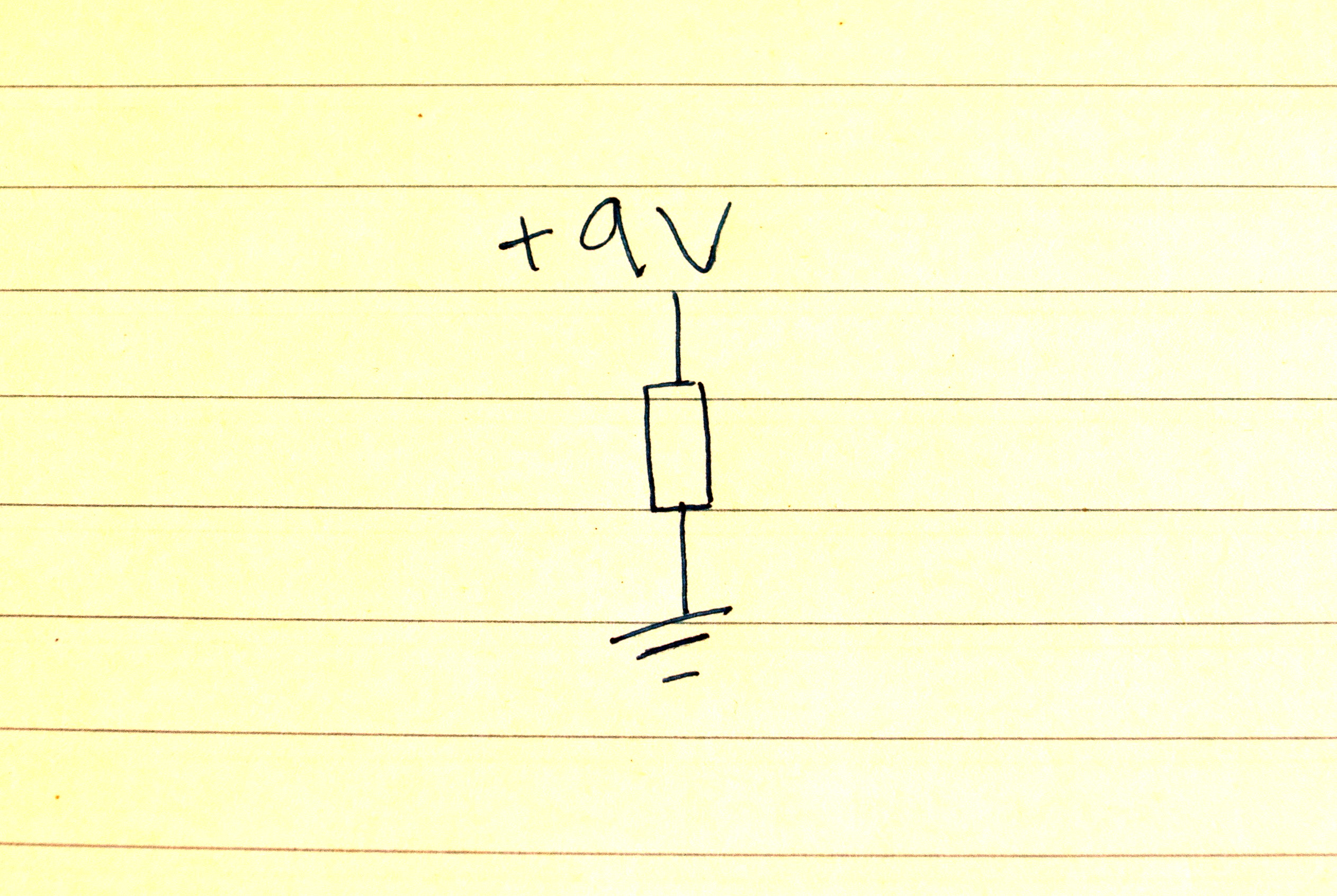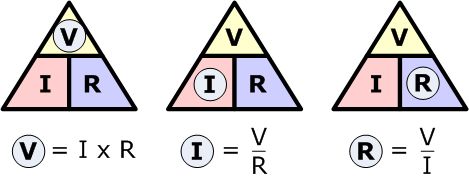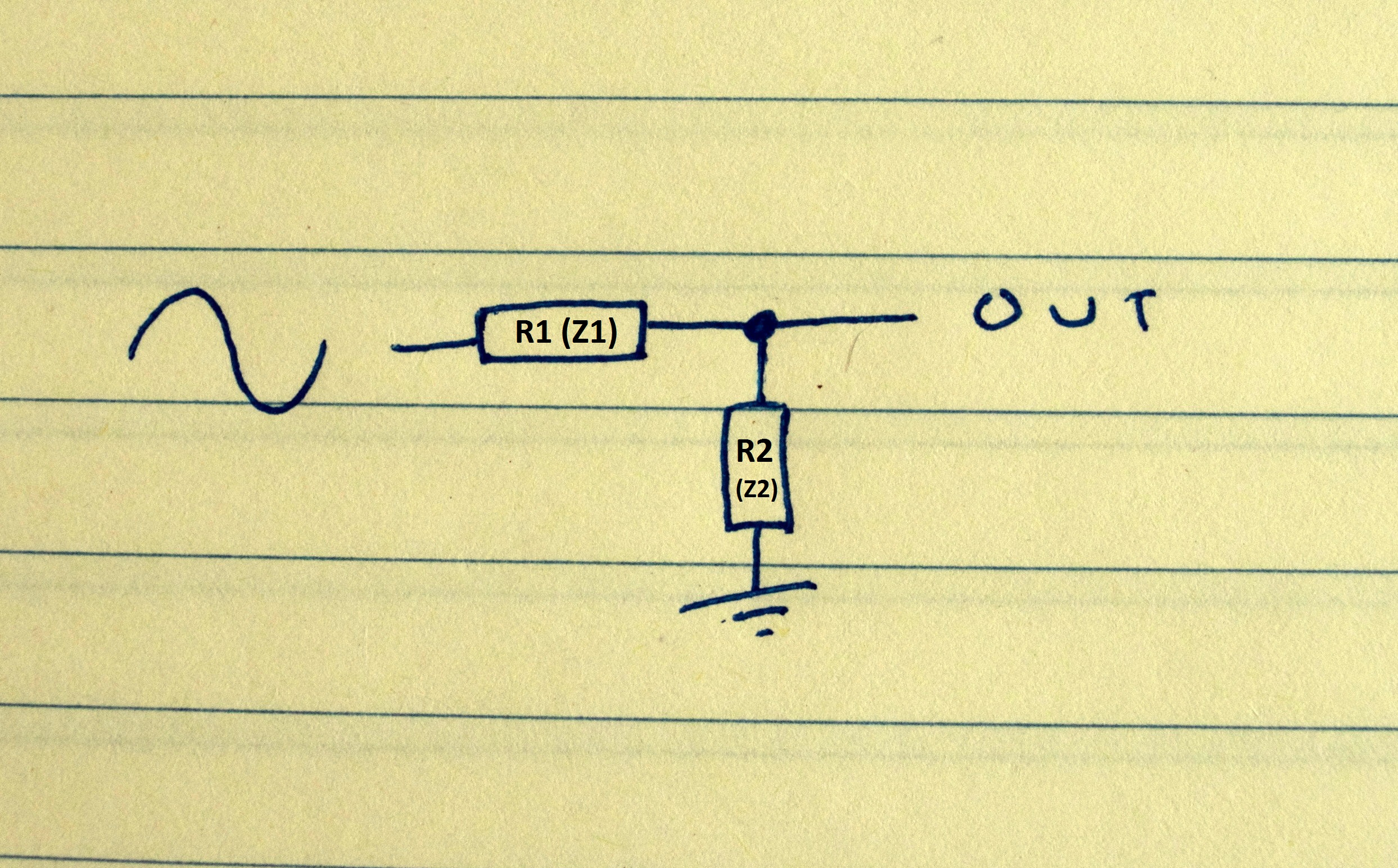# Get outta my way! Understanding Impedance!

###### * This series of ‘theory stuff’ posts is meant to introduce some of the basic science behind electronics. I’m going to avoid all but the simplest of maths. Just to point out, in simplifying, I’m basically telling some small fibs, but I’m doing it for the best of reasons: to help you grasp the concept.*

Impedance is a little tricky, but not as tricky as people make out.

Let’s start with resistors, and let’s go back to DC whilst we’re at it.If we put a resistor in between voltage and ground as a ‘load’, we’ll see that the entire voltage is dropped across that one resistor, and it’s doing its job resisting right? Ok let’s prove that with Ohm’s Law (if you’re going to be making, modding or fixing pedals then you’ll need to know Ohm’s Law and it’s very simple).V = voltage (measured in volts)

R = resistance (measured in ohms – Ω)

I = current (measured in Amperes or Amps)

Current is the flow of electrons. Also you may have noticed that we use ‘I’ instead of ‘C’ for current. ‘I’ stands for intensity. We use ‘C’ for capacitance, but we don’t need to talk about that yet.

As long as we have two of the three we can work out the other. So…

Let’s say we are using a 9V supply.

Let’s also say we are using a 10k resistor

thusly

9 divided by 10,000 equals 0.0009 Amps

If we change the resistor to a 10M (1,000,000 ohms) then

9 divided by 1,000,000 equals 0.000009 Amps

Oh my! See how the resistor resists! In this case we can see its effect on current.

Yes, very handy indeed. Remember, ground is 0 volts (we use ground as a reference point), so the full 9 volts is being dropped across this single resistor. That doesn’t change regardless of the value of the resistor. As we saw above, the value of the resistor affects how much current flows from voltage to ground.

In this ‘circuit’ the single resistor is a load. Never connect a the positive terminal of a voltage source directly to ground without a load. Bad things happen if you do. Things explode, fly off and set your groin on fire. If you’re really curious about connecting the positive terminal to ground put your tongue across the two terminals on a 9V battery. Just don’t get addicted. 1

OK, so… …back to the task at hand. If we give that resistor a friend we have a voltage divider. Like this!Now we have a voltage divider. We know that the two friendly resistors together will drop 9V. We don’t need Ohm’s Law here, because we’re not dealing with current at all. But how do we know how much of the 9V is dropped across each. Well, it’s actually remarkably simple. The amount of voltage we see at the output is a ratio of the two resistors.

So, if R1 and R2 are the same value, we’ll see half the supply voltage (which is 4.5V), which means that the other half is going straight to ground.

If R1 is bigger, we’ll see a small voltage at the output, which means most is being shunted to ground.

If R2 is bigger we’ll see a bigger voltage at the output. This means less than half the voltage applied will go straight to ground via R2.

Still with me?

Now if we think of impedance as series resistance for AC signals and we redraw that voltage divider, we’re getting much closer to what we need to know about input and output impedance. One important note on impedance is that unlike resistance it is not equal across all frequencies.As I mentioned earlier, pickups are inductors. Inductors carry an impedance of their own. Let’s picture R1 (actually we use ‘Z’ to symbolise impedance) as the output impedance from a pedal or from a pickup. R2 (Z2) is the input impedance of the next pedal in the chain.

I’m sure you’re already well ahead of me on this, but yeah, based on the ratio of Z1 and Z2 a certain amount of our guitar signal is going to ground, from whence it never shall return. Obviously we want to keep as much of our signal as possible, especially if you have one of them expensive nice sounding fancy guitars. Sacrificing part of your signal to the god of impedance just to be able to play electronified guitar in the first place is ultimately worth it, it’s something we have to accept, but if you’re into making or even just playing through pedals, it’s something you should probably understand.

As a general rule, it’s best to make sure that the input impedance is ten times bigger than the output impedance. Input impedances ideally will be 1MΩ or above, output impedance should be 100kΩ or below. Obviously the higher the input impedance, and the lower the output impedance the better. A ratio of 10:1 means that roughly 90% of the guitar signal is reaching its destination. That’s not bad. Also so you know, it’s the top end frequencies that usually get lost.

Guitar cables also carry a certain amount of impedance. Now imagine playing through a long lead. The longer the lead the more impedance, which is why in the modern world of true bypass effects, talk of buffers is the (relatively) new big talking point.

Tune in next time for more on buffers and other things starting with the letter B.

1 9V isn’t enough to do anything particularly bad. If you’re messing about on the breadboard, the worst that might happen is you blow up a transistor, or you release the magic smoke. BUT REMEMBER! If you’re using a 9V supply, that thing is plugged into the wall, and mains voltage ain’t nothing to piss on with, or piss on.